# Math in Focus Grade 6 Chapter 13 Answer Key Introduction to Statistics

This handy Math in Focus Grade 6 Workbook Answer Key Chapter 13 Introduction to Statistics detailed solutions for the textbook questions.

## Math in Focus Grade 6 Course 1 B Chapter 13 Answer Key Introduction to Statistics

### Math in Focus Grade 6 Chapter 13 Quick Check Answer Key

Complete. Use the data in the line plot.

The line plot shows the weight, in pounds, of cartons of apples in a store. Each x represents 1 carton of apples.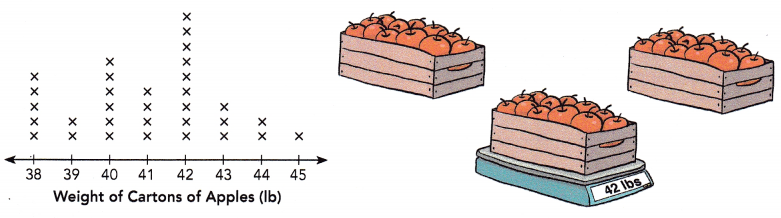Question 1.
What is the weight of the lightest carton of apples?
45 lb
Explanation:
The line plot shows the weight, in pounds, of cartons of apples in a store.
Each x represents 1 carton of apples.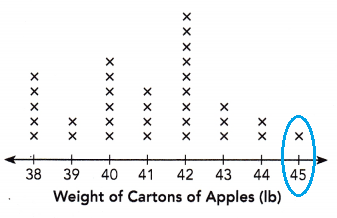So, the weight of one apple carton box (x) = 45 lb
= 45 lb

Question 2.
What is the weight of the heaviest carton of apples?
378 lb
Explanation:
The line plot shows the weight, in pounds, of cartons of apples in a store.
Each x represents 1 carton of apples.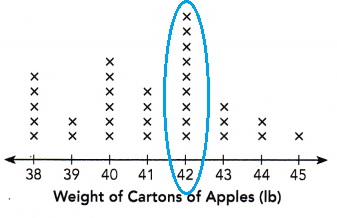9 apple carton box X 45 lb.
The weight of the heaviest carton of apples is = 378 lb

Question 3.
What is the difference in weight between the heaviest carton of apples and the lightest carton of apples?
333 lb
Explanation:
We know from the above questions that the weight of the heaviest carton of apples = 378 lb
The weight of the lightest carton of apples = 45 lb
The difference in weight between the heaviest carton of apples and the lightest carton of apples,
378 – 45 = 333 lb

Question 4.
How many cartons weigh more than 41 pounds?
15 cartons weigh more than 41 pounds.
Explanation:
The line plot shows the weight, in pounds, of cartons of apples in a store.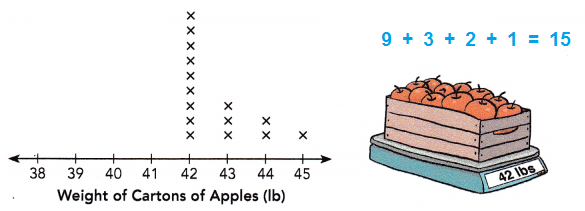9 cartons weigh 42 pounds
3 cartons weigh 43 pounds
2 cartons weigh  44 pounds
1 cartons weigh  45 pounds
9 + 3 + 2 + 1 =  15
15 cartons weigh more than 41 pounds.

Question 5.
How many cartons weigh less than 40 pounds?
7 cartons weighs less than 40 pounds.
Explanation:
The line plot shows the weight, in pounds, of cartons of apples in a store.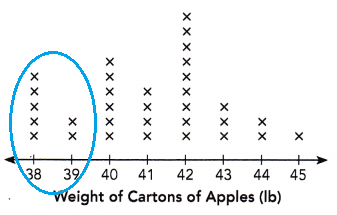5 cartons weigh  38 pounds.
2 cartons weigh  39 pounds.
5 + 2 =  7
So, 7 cartons weigh less than 40 pounds.

Question 6.
How many cartons weigh 44 pounds each?
2 cartons weighs 44 pounds each.
Explanation:
The line plot shows the weight, in pounds, of cartons of apples in a store.
So, 2 cartons weighs 44 pounds each.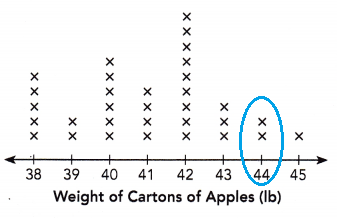Question 7.
How many cartons are there in all?
32 cartons are there in all.
Explanation:
The line plot shows the weight, in pounds, of cartons of apples in a store.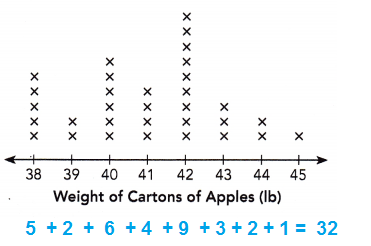So, 32 cartons are there in all.

Question 8.
How many times as many cartons of apples weigh 40 pounds as the number of cartons of apples that weigh 43 pounds?
2 times.
Explanation:
cartons of apples weigh 40 pounds is 6 cartons.
cartons of apples that weigh 43 pounds is 3 cartons.
Number of times as many cartons of apples weigh 40 pounds as the number of cartons of apples that weigh 43 pounds = 6/3 = 2 times.

Question 9.
The ratio of the number of cartons of apples that weigh 42 pounds to the ‘ number of cartons of apples that weigh 40 pounds is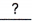.
3 : 2
Explanation:
cartons of apples weigh 42 pounds is 9 cartons.
cartons of apples that weigh 40 pounds is 6 cartons.
Ratio of both the cartons = 9 : 6
By simplifying we get 3 : 2
So, the ratio of the number of cartons of apples that weigh 42 pounds to the ‘ number of cartons of apples that weigh 40 pounds is 3 : 2

Question 10.
The number of cartons of apples that weigh 41 pounds is% of the total number of cartons of apples.